Solution by the quadratic formulaCustom SearchSOLUTION BY THE QUADRATIC FORMULA The quadratic formula is derived by applying the process of completing the square to solve for x in the general form of the quadratic equation, ax2 + bx + c = 0. Remember that the general form represents every possible quadratic equation. Thus, if we can solve this equation for x, the solution will be in terms of a, b, and c. To solve this equation for x by completing the square, we proceed as follows: 1. Subtract the constant term, c, from both members. ax2 + bx + c = 0 ax2 + bx = -c 2. Divide all terms by a so that the coefficient of the x2 term becomes unity.3. Add the square of one-half the coefficient of the x term b/a, to both members.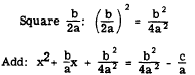4. Factor the left member and simplify the right member.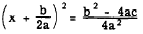5. Take the square root of both members.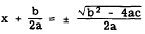6. Solve for x.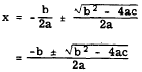Thus, we have solved the equation representing every quadratic for its unknown in terms of its constants a, b, and c. Hence, in a given quadratic we need only substitute in the expressionthe values of a, b, and c, as they appear in the particular equation, to derive the roots of that equation. This expression is called the QUADRATIC FORMULA. The gene r a 1 quadratic equation, ax2 + bx + c = 0, and the quadratic formula should be memorized. Then, when a quadratic cannot be solved quickly by factoring, it may be solved at once by the formula. EXAMPLE: Use the quadratic formula to solve the equation         x2 + 30 - 11x = 0. SOLUTION : 1. Set up the equation in standard form.         X2 - 11x + 30 = 0     Then a (coefficient of x2) = 1               b (coefficient of x) = -11               c (the constant term) = 30 2. Substituting,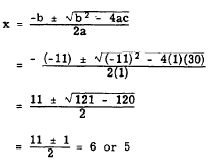3. Checking:EXAMPLE: Find the roots ofSubstituting into the quadratic formula givesThe two roots are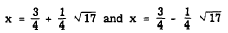These roots are irrational numbers, since the radicals cannot be removed. If the decimal values of the roots are desired, the value of the square root of 17 can be taken from appendix I of this course. Substituting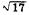= 4.1231 and simplifying gives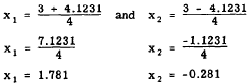In decimal form, the roots of 2x2 - 3x -1 = 0 to the nearest tenth are 1.8 and -0.3. Notice that the subscripts, 1 and 2, are used to distinguish between the two roots of the equation. The three roots of a cubic equation in x might be designated x1, x2, and x1. Sometimes the letter r is used for root. Using r, the roots of a cubic equation could be labeled r1, r2, and r3. Checking: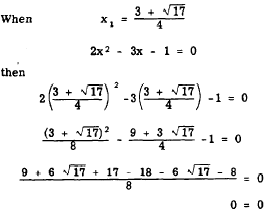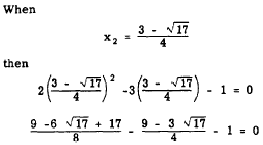Multiplying both members of the equation by 8, the LCD, we have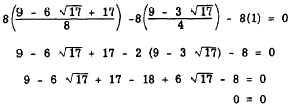Practice problems. Use the quadratic formula to find the roots of the following equations: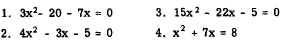Answers: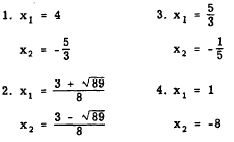Integrated Publishing, Inc. - A (SDVOSB) Service Disabled Veteran Owned Small Business